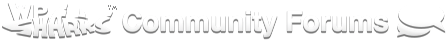# Fixed EOT Issues

Hi,

I am trying to get a Fixed EOT for our membership. All of our memberships end on June 1st. We do allow early renewals. We are using the PayPal forms currently.

I found this article on S2Member.com. However, when I add the ’ rp="[php]echo esc_attr(\$days_until_fixed_eot_time);[/php]" ’ attribute to my shortcode I get this error on the page “Invalid form configuration. Missing “rp” attribute. The Regular Period. Must be >= 1.”

Thanks.

Are you using the ezPHP plugin to do this, as the article recommends?

Yes, I am. You cannot see the PHP code, which normally would be seen without the ezPHP. The only error I get is saying that the S2Member short code is missing the “rp” attribute, which is where one part of the php is.

Where’s the code that provides the values for the variables?

That code is shown in the sample as:

``````[php]
\$now = strtotime('now'); // The time now (in seconds).
\$fixed_eot_time = strtotime('31 October 2025'); // Future time (in seconds).
\$days_until_fixed_eot_time = round((\$fixed_eot_time - \$now) / DAY_IN_SECONDS);
// ↑ This is where we calculate the number of days until an EOT should occur.
[/php]
``````

I am afraid I do not have much knowledge on programming. This is the full example of the code that the article has:

[php]
\$now = strtotime(‘now’); // The time now (in seconds).
\$fixed_eot_time = strtotime(‘31 October 2025’); // Future time (in seconds).
\$days_until_fixed_eot_time = round((\$fixed_eot_time - \$now) / DAY_IN_SECONDS);
// ↑ This is where we calculate the number of days until an EOT should occur.
[/php]

[s2Member-Pro-PayPal-Form level=“1” desc="\$0.01 USD / Access Until October 31st, 2025" ra=“0.01” rr=“BN” rt=“D” rp="[php]echo \$days_until_fixed_eot_time;[/php]" /] - This part has been shortened

However, when using this code (with my pro form shortcode) I keep getting that error? Does this code require PayPal Pro to work (not get that error)? If so, is there no way to do it with the pro-forms and a normal PayPal business account?

Remove the “” around the PHP code! I should have caught that the first time around.

I removed the “” around the php in the shortcode, but I am still getting the same error.

Try this:

[php]
\$now = strtotime(‘now’); // The time now (in seconds).
\$fixed_eot_time = strtotime(‘31 October 2025’); // Future time (in seconds).
\$days_until_fixed_eot_time = round((\$fixed_eot_time - \$now) / DAY_IN_SECONDS);
echo sprintf(’[s2Member-Pro-PayPal-Form level=“1” desc="\$0.01 USD / Access Until October 31st, 2025" ra=“0.01” rr=“BN” rt=“D” rp="%d" /]’, \$days_until_fixed_eot_time);
[/php]

Would I need to put my entire S2Member short code in or just the shortened one you used? Do I still need the php code in the form shortcode? Thanks.

My entire shortcode: [s2Member-Pro-PayPal-Form level=“2” ccaps="" desc=“Frisco HIS Membership - \$30.00” ps=“paypal” lc="" cc=“USD” dg=“0” ns=“1” custom=“friscohis.org” ta=“0” tp=“0” tt=“D” ra=“30.00” rp=“1” rt=“Y” rr=“BN” rrt="" rra=“2” accept=“paypal,visa,mastercard,amex,discover,maestro,solo” accept_via_paypal=“paypal” coupon="" accept_coupons=“0” default_country_code="" captcha=“0” /]

When I inserted this code:

[php]
\$now = strtotime(‘now’); // The time now (in seconds).
\$fixed_eot_time = strtotime(‘1 June 2017’); // Future time (in seconds).
\$days_until_fixed_eot_time = round((\$fixed_eot_time - \$now) / DAY_IN_SECONDS);
echo sprintf(’[s2Member-Pro-PayPal-Form level=“2” ccaps="" desc=“Frisco HIS Membership - \$30.00” ps=“paypal” lc="" cc=“USD” dg=“0” ns=“1” custom=“friscohis.org” ta=“0” tp=“0” tt=“D” ra=“30.00” rr=“BN” rt=“D” rp="%d" rrt="" rra=“2” accept=“paypal,visa,mastercard,amex,discover,maestro,solo” accept_via_paypal=“paypal” coupon="" accept_coupons=“1” default_country_code="" captcha=“0” /]’, \$days_until_fixed_eot_time);
[/php]

I still had the same error.

Are you using any kind of page builder - especially Visual Composer?

Yes, we have Visual Composer, but the code is being placed in the normal Wordpress box using the text tab.

Try this:

``````[php]
\$now = strtotime( 'now' ); // The time now (in seconds).
\$fixed_eot_time = strtotime( '1 June 2017' ); // Future time (in seconds).
\$days_until_fixed_eot_time = round( ( \$fixed_eot_time - \$now ) / DAY_IN_SECONDS );
\$level = do_shortcode( '[s2Member-Pro-PayPal-Form level="2" ccaps="" desc="Frisco HIS Membership - \$30.00" ps="paypal" lc="" cc="USD" dg="0" ns="1" custom="friscohis.org" ta="0" tp="0" tt="D" ra="30.00" rr="BN" rt="D" rp="%d" rrt="" rra="2" accept="paypal,visa,mastercard,amex,discover,maestro,solo" accept_via_paypal="paypal" coupon="" accept_coupons="1" default_country_code="" captcha="0" /]' );
echo sprintf( \$level, \$days_until_fixed_eot_time );
[/php]
``````

You might need to put `echo` before `do_shortcode`.

I have had zero luck using any plugin to run PHP code with Visual Composer. It does not make any difference if you place the code directly in the text editor. The failures I’ve had have been themes that come with VC bundled. Are you using VC as a stand-alone plugin or did it come with your theme?

It came with the theme.

I tried the code. I received the same error when pasted as is. However, when I put the echo before the do_shortcode the form disappeared completely.

Well, I was just trying to make your code make sense.

But if you look properly at the KB article to which you referred at the beginning (and which I have now done), you’ll see that you haven’t followed it at all.

This is how it says to do what you want:

``````[php]
\$now = strtotime( 'now' ); // The time now (in seconds).
\$fixed_eot_time = strtotime( '1 June 2017' ); // Future time (in seconds).
\$days_until_fixed_eot_time = round( ( \$fixed_eot_time - \$now ) / DAY_IN_SECONDS );
[/php]

[s2Member-Pro-PayPal-Form level="2" ccaps="" desc="Frisco HIS Membership - \$30.00" ps="paypal" lc="" cc="USD" dg="0" ns="1" custom="friscohis.org" ta="0" tp="0" tt="D" ra="30.00" rr="BN" rt="D" rp="[php]echo \$days_until_fixed_eot_time;[/php]" rrt="" rra="2" accept="paypal,visa,mastercard,amex,discover,maestro,solo" accept_via_paypal="paypal" coupon="" accept_coupons="1" default_country_code="" captcha="0" /]
``````

Unsurprisingly, it works. I have just tested it.

1 Like

I’m getting the same issue. I followed the solution but am getting the same error.

“Invalid form configuration. Missing “rp” attribute. The Regular Period. Must be >= 1.”

Does anyone have suggestions?

This is the code I am using:

[php]\$now = strtotime(‘now’); // The time now (in seconds). \$fixed_eot_time = strtotime(‘31 March 2021’); // Future time (in seconds). \$days_until_fixed_eot_time = round((\$fixed_eot_time - \$now) / DAY_IN_SECONDS); [/php]

[s2Member-Pro-Stripe-Form level=“1” ccaps="" desc="\$35 USD - 2020 Season, ending March 31 2021" cc=“USD” custom=“[www.phillymtcc.org](http://www.phillymtcc.org/)” ta=“0” tp=“0” tt=“D” ra=“35” rr=“BN” rt=“D” rp="[php]echo esc_attr(\$days_until_fixed_eot_time); [/php]" coupon="" accept_coupons=“0” default_country_code=“US” captcha=“0” /]